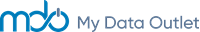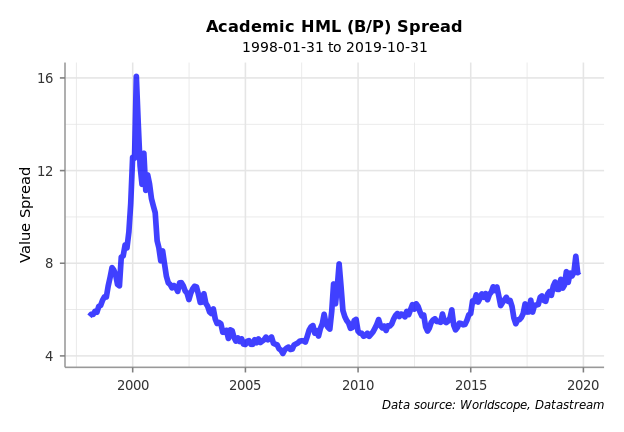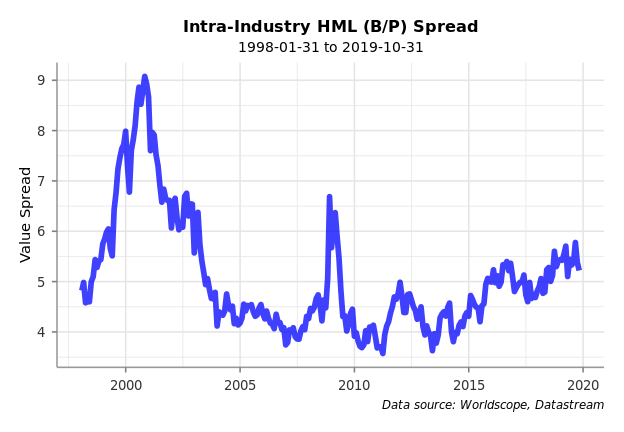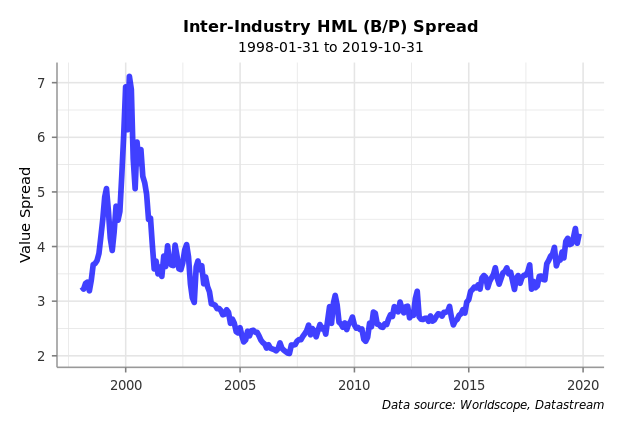# Introduction

In a recent blog post1, Cliff Asness, AQR’s founder, describes different ways to measure the “value of value,” defined as the spread of a value factor between the cheapest and most expensive stocks, and he shows that the current value spread is at a historically high level.

He describes three common methods for measuring the value spread:

1. Academic HML, which only uses book-to-price for the value factor
2. Four-Factor Value Composite, which uses a weighted combination of book-to-price, historical EPS-to-price, forecast EPS-to-price, and sales-to-price
3. AQR’s Value Composite, which is a proprietary composite of 25 factors

He makes the case that due to the spread being so high it is a good time to “sin” a little. It’s a good piece, and we recommend you read it.

For this demonstration, we will show how one can approximately reproduce the first method, Academic HML, using the MDO platform. We say approximately because we use different data sources, securities universe, industry classifications, etc. than the academics, but we get close enough.

# Methodology

First, we’ll create our universe. We’ll start with all the securities in the Datastream universe and filter down to just U.S. companies.

library(mdo.data)
library(mdo.factors)
library(data.table)
library(ggplot2)

startDate <- '1998-01-31'
endDate <- '2019-10-31'
day <- DateRange(startDate = startDate, endDate = endDate, periodType = 'month', dom = 31)

# Create initial universe
security <- DimensionUniverse(items = "DS_SECURITY")
PlugData(security, items = c("DS_REGION", "DS_CMP_COUNTRY"), update = T)
security <- security[DS_REGION == "USA" & DS_CMP_COUNTRY == "USA", security]
universe <- CustomUniverse(day = day, security = security)

Next, we’ll retrieve some basic security and pricing data and further filter down our universe to those securities that trade on the NYSE, NASDAQ, and AMEX stock exchanges.

# Get security and sector info
PlugData(universe = universe, items = c("DS_EXCHANGE", "DS_SECTYPE", "DS_SEC_NAME",
"DS_IS_MAJOR", "RDC_TRBC_ECON_DESC", "RDC_TRBC_BUS_DESC", "RDC_TRBC_INDGRP_DESC"),
update = TRUE)

# Get pricing data
PlugData(universe = universe, items = c("DS_CLOSE", "DS_MKTCAP"),
recent = -10, units = "E", update = TRUE)

# Filter out securities
universe <- universe[DS_IS_MAJOR == "Y"]
universe <- universe[!(is.na(DS_CLOSE) | is.na(DS_MKTCAP))]
universe <- universe[(DS_EXCHANGE %in% c("NEW YORK SE(COMPOSITE)", "NASDAQ(COMPOSITE)",
"NYSE American")) & (DS_SECTYPE == "Ordinary Share")]

Next, we’ll add our book-to-price factor, MDQ_BOOK_TO_PRICE, to the universe. This factor (along with 100+ other quantitative factors) comes pre-loaded with the mdo.factors package. It is defined as the book value per share from Worldscope divided by the most recent price from Datastream. Note: We align the data so that we only retrieve the book value per share that was known at the time to avoid look-ahead bias.

# Get factor data
universe <- as.universe(universe, dimensions = c("day", "security"))
QFData(universe, factors = "MDQ_BOOK_TO_PRICE", update = T)

The following steps will remove securities with a negative book value, select the largest 3000 stocks on each month-end date, and treat outliers by winsorizing the bottom and top 1% of values.

# Exclude stocks with negative fundamentals
universe <- universe[(MDQ_BOOK_TO_PRICE > 0)]

# Select largest securities
universe <- universe[order(-DS_MKTCAP), .SD[1:3000], by = day]

# Winsorize outliers
universe[, VALUE := InterQtileRange(MDQ_BOOK_TO_PRICE, lower = 0.01,
upper = .99, times = 1, replace = T), by = day]

There are three variations of the Academic HML that we will now re-create:

This variation deciles the book-to-price across the entire universe on each month-end date. It then takes the weighted-average book-to-price of those securities in the top three deciles (i.e., cheapest 30% of stocks) and divides by the weighted-average book-to-price of those securities in the bottom three deciles (i.e., most expensive 30% of stocks).

data <- copy(universe)

# Decile the factor values
data[, VALUE_Q := MDOQuantile(VALUE, n = 10), by = 'day']

cheap <- data[VALUE_Q <= 3, .(cheap = sum(DS_MKTCAP / sum(DS_MKTCAP) * VALUE)), by = day]
expensive <- data[VALUE_Q >= 8, .(expensive = sum(DS_MKTCAP / sum(DS_MKTCAP) * VALUE)), by = day]
spread <- cheap[expensive, on = "day"]
spread[, spread := cheap / expensive]In this variation, we decile the book-to-price within the universe by industry on each month-end date to keep things industry-neutral. In this example, we group by the Thomson Reuters Business Classification industry group item, RDC_TRBC_INDGRP_DESC, but you could use the GICS classification from S&P or MSCI, if you are licensed.

data <- copy(universe)

# Decile the factor values by industry
data[, VALUE_Q := MDOQuantile(VALUE, n = 10), by = c("day", "RDC_TRBC_INDGRP_DESC")]

cheap <- data[VALUE_Q <= 3, .(cheap = sum(DS_MKTCAP / sum(DS_MKTCAP) * VALUE)), by = day]
expensive <- data[VALUE_Q >= 8, .(expensive = sum(DS_MKTCAP / sum(DS_MKTCAP) * VALUE)), by = day]
spread <- cheap[expensive, on = "day"]
spread[, spread := cheap / expensive]This variation calculates the book-to-price at the industry-level and deciles across industries on each month-end date. The weighted-average book-to-price of those industries in the top three deciles are divided by the weighted-average book-to-price of those industries in the bottom three deciles.

data <- copy(universe)

# Calculate the B/P of each industry
INDUSTRY_BP <- data[, .(INDUSTRY_MKTCAP = sum(DS_MKTCAP),
VALUE = sum(DS_MKTCAP / sum(DS_MKTCAP) * VALUE)), by = c("day", "RDC_TRBC_INDGRP_DESC")]

INDUSTRY_BP[, VALUE_Q := MDOQuantile(VALUE, n = 10), by = c("day")]

spread[, spread := cheap / expensive]